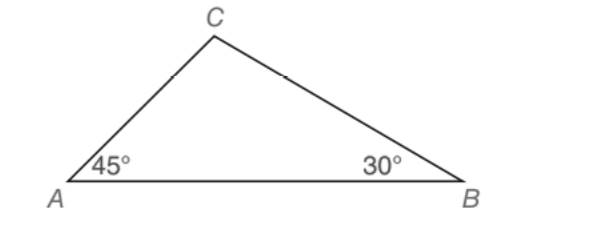Chapter 5.5, Problem 33EElementary Geometry For College St...

7th Edition
Alexander + 2 others
ISBN: 9781337614085

Solutions

Chapter
SectionElementary Geometry For College St...

7th Edition
Alexander + 2 others
ISBN: 9781337614085
Textbook Problem

In Exercises 27 to 33, give both exact solutions and approximate solutions to two decimal places. Given: ∆ A B C with m ∠ A = 45 ° , m ∠ B = 30 ° , and B C = 12 Find: A B (HINT: Use altitude C D ¯ from C to A B ¯ as an auxiliary line)To determine

To find:

AB of the ABC with mA=45°, mB=30° and BC=12.

Explanation

Approach:

For a right triangle, for which the measure of the interior angles 30°, 60°, and 90°; if ‘a’ is the length of measure of the shorter leg; opposite to the angle 30°, then the length of the other two sides is given by

Length of the longer leg (opposite to 60°) =a3

Length of the hypotenuse (opposite to 90°)=2a.

In general

Length of the longer leg =3× (Length of the shorter leg)

Length of the hypotenuse =2× (Length of the shorter leg)

Approach:

For a right triangle for which the measure of the interior angles 45°, 45°, and 90°, if ‘a’ is the length of measure of one of the leg; opposite to the angle 45°, then the length of the other two sides is given by

Length of the other leg =a

Length of the hypotenuse =a2

In general

Length of the legs are equal.

Length of the hypotenuse =2× (Length of one of the legs)

Calculation:

Given,

ABC with

mA=45°,

mB=30° and

BC=12.

Let us find the third angle C of the ABC.

By the property that the sum of all the three interior angle of a triangle is 180°.

A+B+C=180°

45°+30°+C=180°

75°+C=180°

C=105°

Draw the altitude CD¯ from C to AB¯, which divides the ABC into two right triangles ACD and right BCD and also divides the angle at the vertex C of the ABC into two parts.

One part of the angle C in the right ACD is 45° and the other part should be 60°, which lies in the right BCD such that the sum of all the three interior angles in both the right ACD and in the right BCD become 180°

Still sussing out bartleby?

Check out a sample textbook solution.

See a sample solution

The Solution to Your Study Problems

Bartleby provides explanations to thousands of textbook problems written by our experts, many with advanced degrees!

Get Started

Evaluate the integral, if it exists. 15dt(t4)2

Single Variable Calculus: Early Transcendentals, Volume I

Evaluate each expression: (8+6)4+8

Elementary Technical Mathematics

Write the sum in expanded form. 8. j=nn+3j2

Single Variable Calculus: Early Transcendentals

True or False: n=1an converges if and only if limnan=0.

Study Guide for Stewart's Single Variable Calculus: Early Transcendentals, 8th